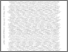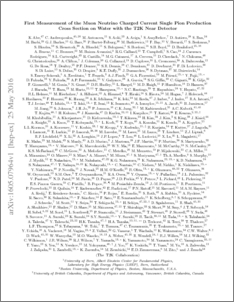# First measurement of the muon neutrino charged current single pion production cross section on water with the T2K near detector

, T2K Collaboration (2017) First measurement of the muon neutrino charged current single pion production cross section on water with the T2K near detector. Physical Review D, 95 (1). ISSN 1550-7998Preview
PDF (1605.07964v1)
1605.07964v1.pdf - Accepted Version

## Abstract

The T2K off-axis near detector, ND280, is used to make the first differential cross section measurements of muon neutrino charged current single positive pion production on a water target at energies ${\sim}0.8$~GeV. The differential measurements are presented as a function of muon and pion kinematics, in the restricted phase-space defined by $p_{\pi^+}>200$MeV/c, $p_{\mu^-}>200$MeV/c, $\cos \theta_{\pi^+}>0.3$ and $\cos \theta_{\mu^-}>0.3$. The total flux integrated $\nu_\mu$ charged current single positive pion production cross section on water in the restricted phase-space is measured to be $\langle\sigma\rangle_\phi=4.25\pm0.48~(\mathrm{stat})\pm1.56~(\mathrm{syst})\times10^{-40}~\mathrm{cm}^{2}/\mathrm{nucleon}$. The total cross section is consistent with the NEUT prediction ($5.03\times10^{-40}~\mathrm{cm}^{2}/\mathrm{nucleon}$) and 2$\sigma$ lower than the GENIE prediction ($7.68\times10^{-40}~\mathrm{cm}^{2}/\mathrm{nucleon}$). The differential cross sections are in good agreement with the NEUT generator. The GENIE simulation reproduces well the shapes of the distributions, but over-estimates the overall cross section normalization.

Item Type:
Journal Article
Journal or Publication Title:
Physical Review D
Subjects:
Departments:
ID Code:
82843
Deposited By:
Deposited On:
14 Nov 2016 11:58
Refereed?:
Yes
Published?:
Published